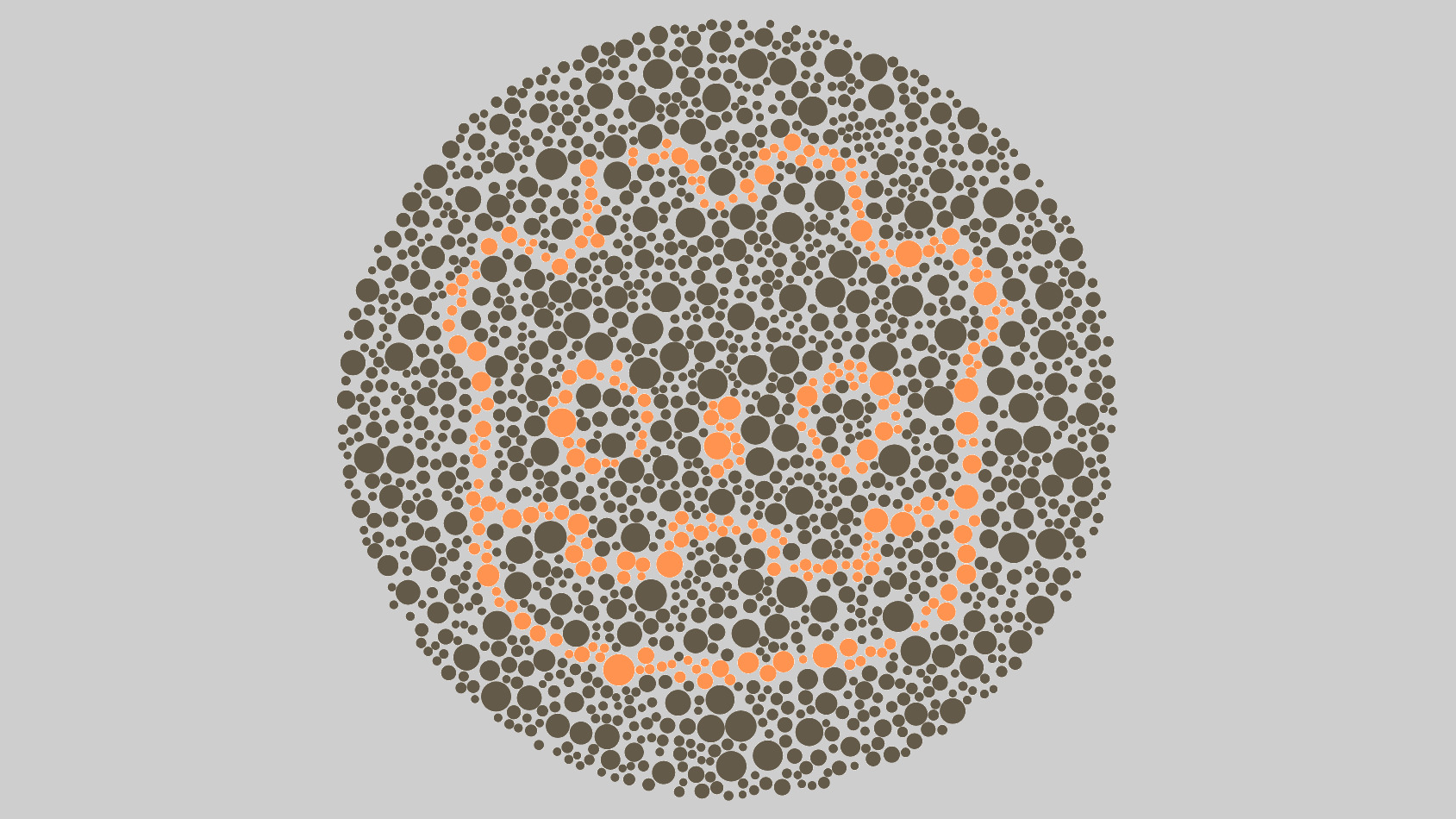This shader can correct for colorblindness, similar to accessibility filters in Windows, MacOS, etc.

This is not a colorblindness simulation shader.

For anyone interested in making an accessible game, beware that a colorblind filter generally only has limited efficiency. It can help if you don’t have time in reviewing your entire game to be accessible, but it’s not an ideal solution. Instead, a better way to create an accessible game is to ensure nothing relies on color alone to be recognized (use shapes, differences in brightness, etc). Still, some color combinations such as yellow and blue work better than others, especially since they also have a significant difference in brightness. (/u/Calinou on Reddit)

Supports protanopia, deuteranopia and tritanopia and has adjustable intensity.

The correction algorithm is taken from Daltonize.org

Image used in screenshots by blende12 at Pixabay

``````/*
* Protanopia (Greatly reduced reds)
* Deuteranopia (Greatly reduced greens)
* Tritanopia (Greatly reduced blues)

The correction algorithm is taken from http://www.daltonize.org/search/label/Daltonize

This shader is released under the CC0 license. Feel free to use, improve and change this shader and consider sharing the modified result.
*/

// Color correction mode
// 0 - Protanopia
// 1 - Deutranopia
// 2 - Tritanopia
uniform int mode : hint_range(0, 2) = 0;

uniform float intensity : hint_range(0.0, 1.0) = 1.0;

void fragment()
{
vec4 tex = texture(SCREEN_TEXTURE, SCREEN_UV);

float L = (17.8824 * tex.r) + (43.5161 * tex.g) + (4.11935 * tex.b);
float M = (3.45565 * tex.r) + (27.1554 * tex.g) + (3.86714 * tex.b);
float S = (0.0299566 * tex.r) + (0.184309 * tex.g) + (1.46709 * tex.b);

float l, m, s;
if (mode == 0) //Protanopia
{
l = 0.0 * L + 2.02344 * M + -2.52581 * S;
m = 0.0 * L + 1.0 * M + 0.0 * S;
s = 0.0 * L + 0.0 * M + 1.0 * S;
}

if (mode == 1) //Deuteranopia
{
l = 1.0 * L + 0.0 * M + 0.0 * S;
m = 0.494207 * L + 0.0 * M + 1.24827 * S;
s = 0.0 * L + 0.0 * M + 1.0 * S;
}

if (mode == 2) //Tritanopia
{
l = 1.0 * L + 0.0 * M + 0.0 * S;
m = 0.0 * L + 1.0 * M + 0.0 * S;
s = -0.395913 * L + 0.801109 * M + 0.0 * S;
}

vec4 error;
error.r = (0.0809444479 * l) + (-0.130504409 * m) + (0.116721066 * s);
error.g = (-0.0102485335 * l) + (0.0540193266 * m) + (-0.113614708 * s);
error.b = (-0.000365296938 * l) + (-0.00412161469 * m) + (0.693511405 * s);
error.a = 1.0;
vec4 diff = tex - error;
vec4 correction;
correction.r = 0.0;
correction.g =  (diff.r * 0.7) + (diff.g * 1.0);
correction.b =  (diff.r * 0.7) + (diff.b * 1.0);
correction = tex + correction;
correction.a = tex.a * intensity;

COLOR = correction;
}``````
###### Tags
colorblind, colorblindness, daltonise, daltonize, deuteranope, deuteranopia, protanope, protanopia, tritanope, tritanopiaThe shader code and all code snippets in this post are under CC0 license and can be used freely without the author's permission. Images and videos, and assets depicted in those, do not fall under this license. For more info, see our License terms.

### Gradient Color Correction for ViewportTextures